# Tridecagon

Regular tridecagon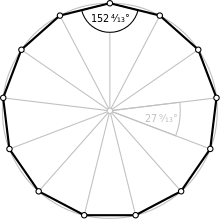A regular tridecagon
Type Regular polygon
Edges and vertices 13
Schläfli symbol {13}
Coxeter diagramSymmetry group Dihedral (D13), order 2×13
Internal angle (degrees) ≈152.308°
Dual polygon Self
Properties Convex, cyclic, equilateral, isogonal, isotoxal

In geometry, a tridecagon or triskaidecagon or 13-gon is a thirteen-sided polygon.

## Regular tridecagon

A regular tridecagon is represented by Schläfli symbol {13}.

The measure of each internal angle of a regular tridecagon is approximately 152.308 degrees, and the area with side length a is given by## Construction

As 13 is a Pierpont prime but not a Fermat prime, the regular tridecagon cannot be constructed using a compass and straightedge. However, it is constructible using neusis, or an angle trisector.

The following is an animation from a neusis construction of a regular tridecagon with radius of circumcircleaccording to Andrew M. Gleason, based on the angle trisection by means of the Tomahawk (light blue).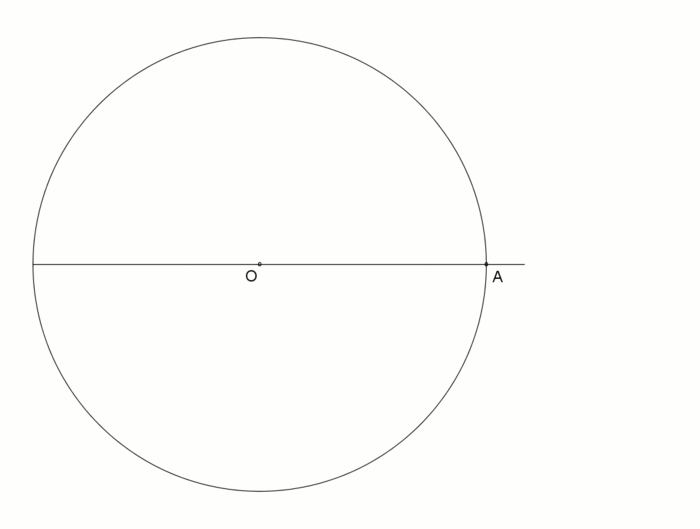A regular tridecagon (triskaidecagon) with radius of circumcircleas an animation (1 min 44 s),
angle trisection by means of the Tomahawk (light blue)

An approximate construction of a regular tridecagon using straightedge and compass is shown here.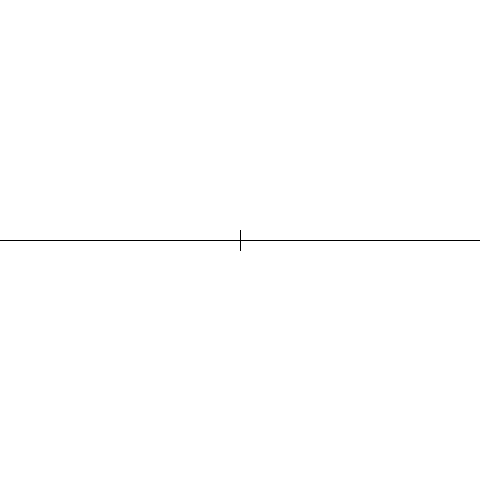Another possible animation of an approximate construction, also possible with using straightedge and compass.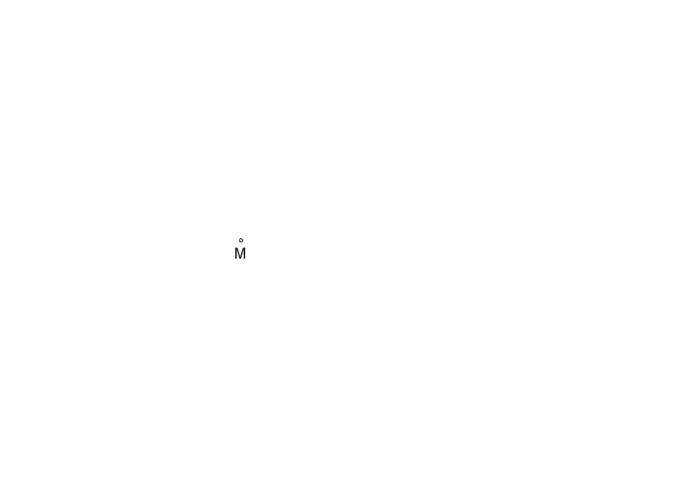Tridecagon, approximate construction as an animation (3 min 30 s)

GeoGebra:BME1 = 27.692307692307764°

GeoGebra: 360° ÷ 13 = 27.69230769230769°

Absolute error ofBME1 ≈ 7.4E-14°

Example to illustrate the error:

At a circumscribed circle radius r = 1 billion km (the light needed for this distance about 55 minutes), the absolute error of the 1st side would be approximately 1 mm.

For details, see: Wikibooks: Tridecagon, construction description (German)

## Symmetry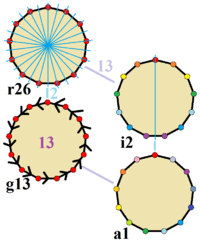Symmetries of a regular tridecagon. Vertices are colored by their symmetry positions. Blue mirrors are drawn through vertices and edge. Gyration orders are given in the center.

The regular tridecagon has Dih13 symmetry, order 26. Since 13 is a prime number there is one subgroup with dihedral symmetry: Dih1, and 2 cyclic group symmetries: Z13, and Z1.

These 4 symmetries can be seen in 4 distinct symmetries on the tridecagon. John Conway labels these by a letter and group order. Full symmetry of the regular form is r26 and no symmetry is labeled a1. The dihedral symmetries are divided depending on whether they pass through vertices (d for diagonal) or edges (p for perpendiculars), and i when reflection lines path through both edges and vertices. Cyclic symmetries in the middle column are labeled as g for their central gyration orders.

Each subgroup symmetry allows one or more degrees of freedom for irregular forms. Only the g13 subgroup has no degrees of freedom but can seen as directed edges.

## Numismatic use

The regular tridecagon is used as the shape of the Czech 20 korun coin.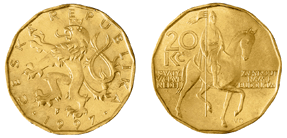## Related polygons

A tridecagram is a 13-sided star polygon. There are 5 regular forms given by Schläfli symbols: {13/2}, {13/3}, {13/4}, {13/5}, and {13/6}.

### Petrie polygons

The regular tridecagon is the Petrie polygon 12-simplex:

A12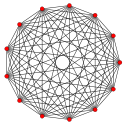12-simplex

## References

1. Gleason, Andrew Mattei (March 1988). "Angle trisection, the heptagon, and the triskaidecagon p. 192–194 (p. 193 Fig.4)" (PDF). The American Mathematical Monthly. 95 (3): 186–194. Archived from the original (PDF) on 2015-12-19. Retrieved 24 December 2015.
2. John H. Conway, Heidi Burgiel, Chaim Goodman-Strauss, (2008) The Symmetries of Things, ISBN 978-1-56881-220-5 (Chapter 20, Generalized Schaefli symbols, Types of symmetry of a polygon pp. 275-278)
3. Colin R. Bruce, II, George Cuhaj, and Thomas Michael, 2007 Standard Catalog of World Coins, Krause Publications, 2006, ISBN 0896894290, p. 81.